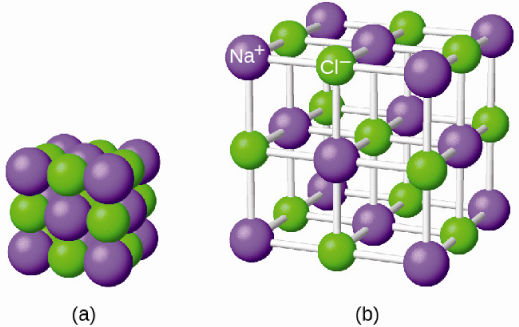# 7.1 Ionic bonding  (Page 2/5)

 Page 2 / 5

As all substances must be electrically neutral, the total number of positive charges on the cations of an ionic compound must equal the total number of negative charges on its anions. The formula of an ionic compound represents the simplest ratio of the numbers of ions necessary to give identical numbers of positive and negative charges. For example, the formula for aluminum oxide, Al 2 O 3 , indicates that this ionic compound contains two aluminum cations, Al 3+ , for every three oxide anions, O 2− [thus, (2 $×$ +3) + (3 $×$ –2) = 0].

It is important to note, however, that the formula for an ionic compound does not represent the physical arrangement of its ions. It is incorrect to refer to a sodium chloride (NaCl) “molecule” because there is not a single ionic bond, per se, between any specific pair of sodium and chloride ions. The attractive forces between ions are isotropic—the same in all directions—meaning that any particular ion is equally attracted to all of the nearby ions of opposite charge. This results in the ions arranging themselves into a tightly bound, three-dimensional lattice structure. Sodium chloride, for example, consists of a regular arrangement of equal numbers of Na + cations and Cl anions ( [link] ).The atoms in sodium chloride (common table salt) are arranged to (a) maximize opposite charges interacting. The smaller spheres represent sodium ions, the larger ones represent chloride ions. In the expanded view (b), the geometry can be seen more clearly. Note that each ion is “bonded” to all of the surrounding ions—six in this case.

The strong electrostatic attraction between Na + and Cl ions holds them tightly together in solid NaCl. It requires 769 kJ of energy to dissociate one mole of solid NaCl into separate gaseous Na + and Cl ions:

$\text{NaCl}\left(s\right)\phantom{\rule{0.2em}{0ex}}⟶\phantom{\rule{0.2em}{0ex}}{\text{Na}}^{\text{+}}\left(g\right)+{\text{Cl}}^{\text{–}}\left(g\right)\phantom{\rule{3em}{0ex}}\text{Δ}H=769\phantom{\rule{0.2em}{0ex}}\text{kJ}$

## Electronic structures of cations

When forming a cation, an atom of a main group element tends to lose all of its valence electrons, thus assuming the electronic structure of the noble gas that precedes it in the periodic table. For groups 1 (the alkali metals) and 2 (the alkaline earth metals), the group numbers are equal to the numbers of valence shell electrons and, consequently, to the charges of the cations formed from atoms of these elements when all valence shell electrons are removed. For example, calcium is a group 2 element whose neutral atoms have 20 electrons and a ground state electron configuration of 1 s 2 2 s 2 2 p 6 3 s 2 3 p 6 4 s 2 . When a Ca atom loses both of its valence electrons, the result is a cation with 18 electrons, a 2+ charge, and an electron configuration of 1 s 2 2 s 2 2 p 6 3 s 2 3 p 6 . The Ca 2+ ion is therefore isoelectronic with the noble gas Ar.

For groups 12–17, the group numbers exceed the number of valence electrons by 10 (accounting for the possibility of full d subshells in atoms of elements in the fourth and greater periods). Thus, the charge of a cation formed by the loss of all valence electrons is equal to the group number minus 10. For example, aluminum (in group 13) forms 3+ ions (Al 3+ ).

how can someone understand chemistry vividly
Maybe by reading proofs or practical work and application in modern world.
Abdelkarim
what is isotopes
whats de shape of water
water has no shape because it's liquid
Wil
water is a shapeless, odourless, colourless and tasteless substance that only takes the shape of its container.
mikefred
i think they're referring to the molecular shape?
It has no shape but takes the shape of the container
what isthe maening pkw
introduction to chemistry for beginner
hi
noble
through out human history
Yes?
Esther
hello
noble
yes
Bol
what
noble
is euglena a unicellular organ
is euglena a unicellular organism
Agio
how is hydrogen can be heated
what is difference between atom and molecule
Atom is the smallest part of matter; it consists of equal number of protons and electrons. It may have neutrons. A molecule is a compound made of atoms covalently bonded.
Abdelkarim
does amoeba has structure
what is the effect of green house on the earth 🌎
what is the effect of green house on the earth 🌎
kolawole
what's an atom?
it's the smallest unit of Matter
Orsine
atom
Eden
smallest part of an element
lydia
also, depending on its (atom's) structure, that is the amount of protons and neutrons and electrons, is the determining factors of what element it is.
Richard
is a smallast particals of an element
Buwembo
it is the smallest part of an element that can take part in a chemical reaction
Ayub
is the smallest part of an element
Jonathan
An atom is the smallest indivisible part of a matter
Oy kl konsa test hay or kitna hay?
differences between solid liquid and gaseous state
modification of John dalton atomic theory
Ochei
the differences between soliq liquid and gas is that in solid the particle are strongly bonded together by forces of cohesion and the particle are not able to move about but only vibrate in a fixed position but in liquid the particle are loosely bond together and the particle are able to move about
kolawole
2.4g of magnesium reacts with 0.3mol of hydrochloric acid write a balanced chemical equation for the reaction. (b)Determine the limiting reactant

#### Get Jobilize Job Search Mobile App in your pocket Now!ByByBy OpenStaxBy Marion CabalfinBy Zarina ChocolateBy Yasser IbrahimBy Candice ButtsBy OpenStaxBy Hope PercleByBy OpenStaxBy Qqq Qqq